CAT  >  Examples: Polygons

# Examples: Polygons Notes | Study Quantitative Aptitude (Quant) - CAT

## Document Description: Examples: Polygons for CAT 2022 is part of Quantitative Aptitude (Quant) preparation. The notes and questions for Examples: Polygons have been prepared according to the CAT exam syllabus. Information about Examples: Polygons covers topics like and Examples: Polygons Example, for CAT 2022 Exam. Find important definitions, questions, notes, meanings, examples, exercises and tests below for Examples: Polygons.

Introduction of Examples: Polygons in English is available as part of our Quantitative Aptitude (Quant) for CAT & Examples: Polygons in Hindi for Quantitative Aptitude (Quant) course. Download more important topics related with notes, lectures and mock test series for CAT Exam by signing up for free. CAT: Examples: Polygons Notes | Study Quantitative Aptitude (Quant) - CAT
 1 Crore+ students have signed up on EduRev. Have you?

Polygons

A closed plane figure made up of several line segments that are joined together is called a polygon. The sides
do not cross each other. Exactly two sides meet at every vertex.

Types of Polygons:

Regular: all angles are equal and all sides are of same length. Regular polygons are both equiangular and
equilateral.

Equiangular: all angles are equal.

Equilateral: all sides are of same length.

Properties of Polygon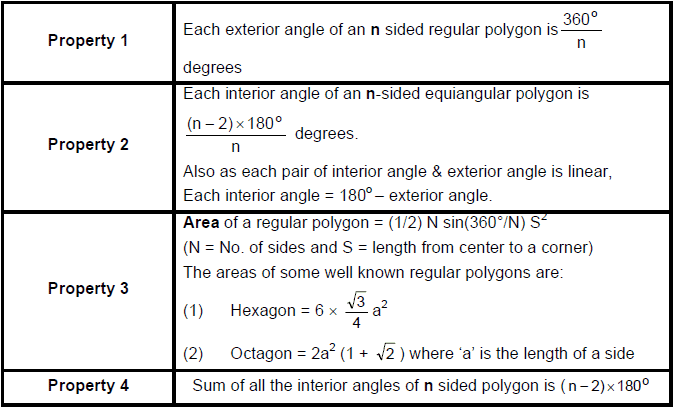A polygon with 4 sides, is a quadrilateral

1. In a quadrilateral, sum of the four interior angles is equal to 360° and also the sum of exterior angles
equal to 360°.

2. On the properties, quadrilaterals have been named differently, as given below.

Trapezium

(a) When one pair of opposite sides is parallel and no condition for other pair of opposite sides, then the

(b) When the non – parallel sides are equal in length then
the trapezium formed is “isosceles trapezium”.

(c) The diagonals of isosceles trapezium are equal in
length but do not bisect.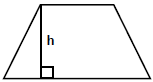Parallelogram

(a) Opposite sides are equals and parallel.

(b) Opposite angles are equal.

(c) Diagonals bisect each other

(d) Each diagonal divides the parallelogram into two triangles of equal area.

(e) Sum of any two adjacent angles is 180°.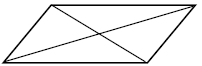Rhombus

(a) All sides are equal, opposite sides are parallel.

(b) Opposite angles are equal.

(c) Diagonals bisect each other at right-angled. But they are not equal.

(d) Each diagonal divides the rhombus into two triangles of equal area.

(e) Area =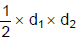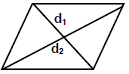Rectangle

(a) Pair of opposite sides equal.

(b) Each angle is 90o.

(c) Diagonals are equal and bisect each other (not at right angles).

(d) Length of diagonal =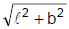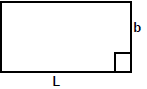Square

(a) All sides are equal and angles equal.

(b) Diagonals equal and bisect at 90O.

(c) When it is inscribed in a circle, the diagonal of square is equal to
the diameter of the circle. But when circle is inscribed in a square,
the side of the square is equal to diameter of the circle.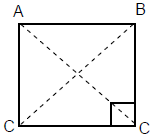Important Results

If we join the mid-point of a quadrilateral we get a parallelogram and the mid-point of parallelogram will give a
rectangle. If we again in join the mid-point of rectangle we get a rhombus and the mid point of rhombus will
give you a square.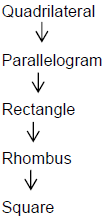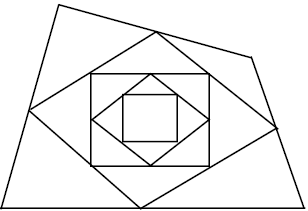Circles

If O is a fixed point in a given plane, the set of points in the plane which are at equal distances from O will
form a circle.

Parts of circle

In the figure below O is the centre of the circle of radius OM and diameter AB. Here diameter is always twice
the radius of the circle. CD is the secant to the circle and cut the circle at two different points. The tangent
PTR touches the circle at one and only one point. Also we have infinite number of tangent on a circle but at a
point there will be one and only one tangent that can be drawn. The chord TS in a circle is the line which
touches the circle at two different points and diameter is the longest chord.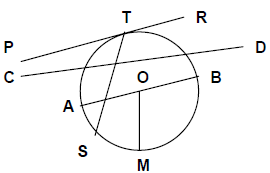Properties of a Circle

1. If two chords of a circle are equal, their corresponding arcs have equal measure.

2. Measurement of an arc is the angle subtended at the centre. Equal arcs subtend equal angles at the
center.

3. A line from centre and perpendicular to a chord bisects the chord.

4. Equal chords of a circle are equidistant from the centre.

5. When two circles touch, their centres and their point of contact are collinear.

6. If the two circles touch externally, the distance between their centres is equal to sum of their radii.

7. If the two circles touch internally, the distance between the centres is equal to difference of their radii.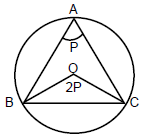8. Angle at the centre made by an arc is equal to twice the angle made by the arc at any point on the
remaining part of the circumference.
Let O be the centre of the circle.
∠BOC = 2 ∠P, when ∠BAC = ∠P

9. If two chords are equal then the arc containing the chords will also be equal.

10. There can be one and only one circle that touches three non-collinear points.

11. The angle inscribed in a semicircle is 90o.

12. If two chords AB and CD intersect externally or internally at P, then
PA × PB = PC × PD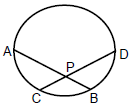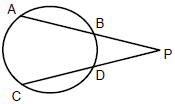14. If PAB is a secant and PT is a tangent, then
PT2 = PA × PB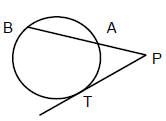15. The length of the direct common tangent (PQ)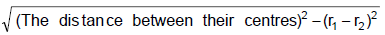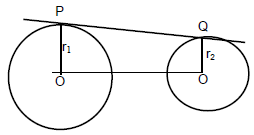16. The length of the transverse common tangent (RS)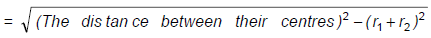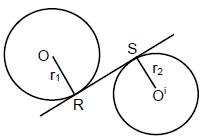If a quadrilateral is inscribed in a circle i.e. all the vertex lies on the circumference of the circle, it is said to be

1. In a cyclic quadrilateral, opposite angles are supplementary.

2. In a cyclic quadrilateral, if any one side is extended, the exterior angle so formed is equal to the interior
opposite angle.

Alternate angle theorem

Angles in the alternate segments are equal.

In the given figure, AC is a Chord touching the circle at points A and C. At point A we have a tangent PAT
making ∠CAT and ∠CAP with the chord AC. In the circle ∠ABC and ∠ADC are two angles in two different
segments.

Here for ∠CAT, the ∠ADC is in alternate segment and for ∠CAP; the ∠ABC is in alternate segment. So
according to the statement of the theorem the pair of these alternate angles are equal to each other.
Then, ∠CAT = ∠ ADC &
∠ PAC = ∠ ABC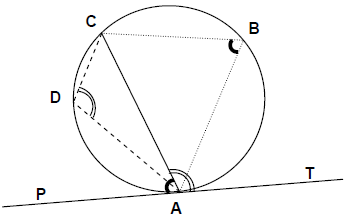The document Examples: Polygons Notes | Study Quantitative Aptitude (Quant) - CAT is a part of the CAT Course Quantitative Aptitude (Quant).
All you need of CAT at this link: CAT

## Quantitative Aptitude (Quant)

163 videos|164 docs|131 tests
 Use Code STAYHOME200 and get INR 200 additional OFF

## Quantitative Aptitude (Quant)

163 videos|164 docs|131 tests

### How to Prepare for CAT

Read our guide to prepare for CAT which is created by Toppers & the best Teachers

Track your progress, build streaks, highlight & save important lessons and more!

,

,

,

,

,

,

,

,

,

,

,

,

,

,

,

,

,

,

,

,

,

;